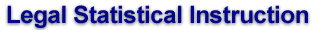Section1: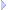2 x 2 TablesChi-SquareDegrees of Freedomp-ValueFlip Coin 100 times
Breast Cancer Example

### Flip each coin 100 times

Now let’s flip the coins 100 times each, and assume the following results: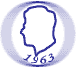Number of HeadsNumber of Tails Totals Coin A 50 50 100 Coin B 70 30 100 Totals 120 20 200

Suppose we again think that the coins have the same probability of heads and tails.

### Question

Can we now reject the hypothesis that the observed difference between the coins occurs by
chance? What does your intuition tell you?

Applying Equation (1) to this table yields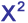= 8.333, with one degree of freedom.

A table of thedistribution shows that there is less than one chance in one hundred of observing such a large difference in the proportion of heads if the same process were governing both coins.

,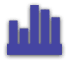Handy chi-square and p-value calculator, developed by Georgetown University

Copyright © 2002 by Theodore Eisenberg & Kevin M. ClermontCornell Law School Cornell University Comments to ted@teddy.law.cornell.edu Last updated: September 2002